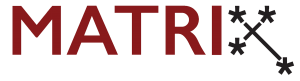Description:

Motivated by the notion of entropy for continuous morphisms in a topological dynamical system, one can similarly define the notion of categorical entropy for endofunctors in triangulated categories. Computing entropy of a given endofunctor can be quite complicated – even in topology, many papers were devoted to introduce different methods of computing entropies for specific morphisms. In many recent works, it was shown that various topological notions have well defined analogies in homological algebra. Guided by these analogies, one can hope to develop methods to compute categorical entropy by emulating methods developed in topology. This symposium aims to gather students from these two seemingly different fields to learn the ideas from topology and translate them into ideas in homological algebra.

The specific goals are: to learn the necessary and related homological algebraic background, learn about dynamical systems in triangulated categories, learn about dynamical systems in topology, and produce methods of computing categorical entropy.

Organisers:
Edmund Heng (Australian National University)
KieSeng Nge (Australian National University)

Contact: If you have any questions, please don’t hesitate to contact the organisers at cat.dynamics2021@gmail.com

Invited Speakers:
Fabian Haiden (Oxford University)
Yu Qiu (Tsinghua University)
TBC

Symposium Structure:

Week 1 – 3 : Introduction and learning week The main aim of this week is to learn the basic concepts of dynamical systems in both topology and triangulated categories. Throughout the week, there will be short talks provided to introduce students to the relevant concepts. Students will be given the relevant papers and notes to read and learn the materials, alongside some assigned problems to work on. Between the talks, there will be discussion sessions where students get to discuss the assigned problems. Students will be
separated into discussion groups according to their mathematical background, with the aim of each group having a good mix of students coming from topological background and homological algebraic background (if numbers allow). There will also be a forum set up throughout the symposium where students can post and answer questions regarding the materials.

Week 4: Online research collaboration week Each group will get to discuss and pick some endofunctors of their interest to work on. Throughout the week, students will work as a group towards computing the categorical entropies of said endofunctors, where they can meet as often as they would like to. Towards the end of the week, each group will be asked to present their progress on the computation, reporting any methods that they have tried to emulate from topology (if any). Students are then encouraged to work
on the problem post the symposium if they would like to extend their results and continue collaborating. This further collaboration will be supported by AMSI and MATRIX.

Registration: